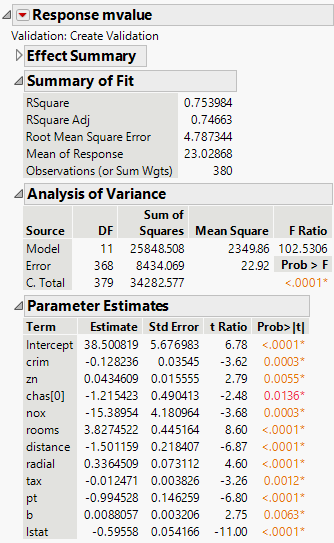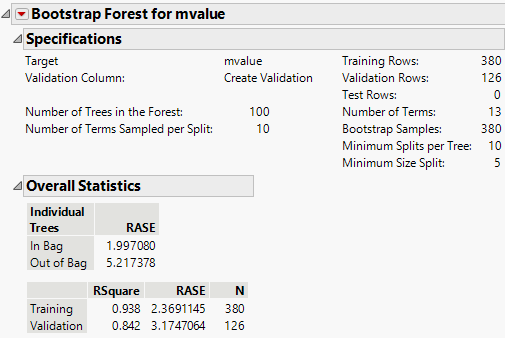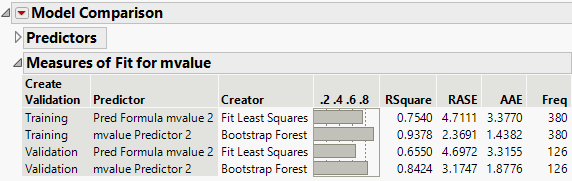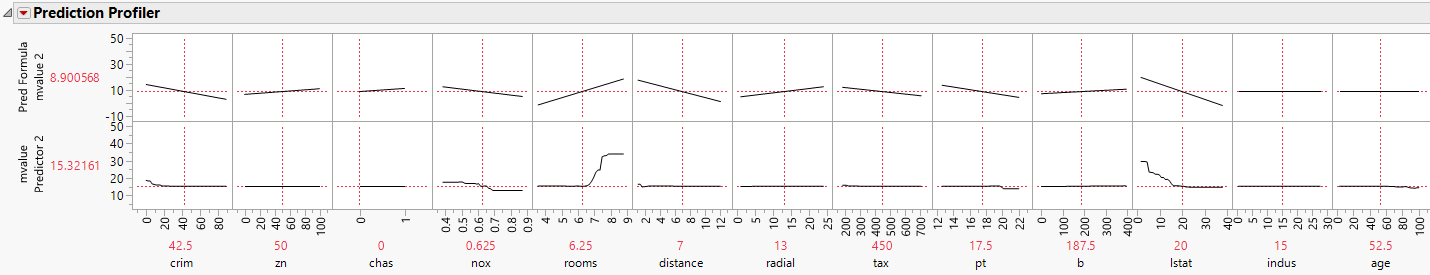Predictive and Specialized Modeling > Model Comparison > Example of Model Comparison
Publication date: 03/23/2021

#Example of Model Comparison

This section provides an example of using the Model Comparison platform. The example uses demographic data to build a model for median home price. A regression model and a bootstrap forest model are compared.

Begin by selecting Help > Sample Data Library and opening Boston Housing.jmp.

#### Create a Validation Column

1. Select Analyze > Predictive Modeling > Make Validation Column.

2. Do not select any columns in the launch window.

This indicates that the platform will create a simple random validation column

3. Click OK.

4. In the box next to New Column Name, type Create Validation.

5. In the box next to Random Seed, enter 1234.

6. Click Go.

A new Validation column is created. The rows assigned a 0 are the training set. The rows assigned a 1 are the validation set.

#### Create the Regression Model and Save the Prediction Formula to a Column

1. Select Analyze > Fit Model.

2. Select mvalue and click Y.

3. Select crim through lstat and click Add.

4. Select Create Validation and click Validation.

5. Select Stepwise in the Personality list.

6. Click the Run button.

7. Select P-value Threshold from the Stopping Rule list.

8. Click the Go button.

9. Click the Run Model button.

Figure 11.2 Fit Model Report10. To save the prediction formula to a column, click the Response red triangle and select Save Columns > Prediction Formula.

#### Create the Bootstrap Forest Model and Save the Prediction Formula to a Column

1. Select Analyze > Predictive Modeling > Bootstrap Forest.

2. Select mvalue and click Y, Response.

3. Select crim through lstat and click X, Factor.

4. Select Create Validation and click Validation.

5. Click OK.

6. Select the Early Stopping check box.

7. Select the Multiple Fits over number of terms check box.

8. Enter 617 in the box next to Random Seed.

9. Click OK.

Figure 11.3 Bootstrap Forest Model10. To save the prediction formula to a column, click the Bootstrap Forest red triangle and select Save Columns > Save Prediction Formula.

#### Compare the Models

1. Select Analyze > Predictive Modeling > Model Comparison.

2. Select the two prediction formula columns and click Y, Predictors.

3. Select Create Validation and click Group.

Tip: If a Group column is not specified, JMP automatically recognizes when the same validation column has been used for all predictors and prompts you to add it as a grouping variable.

4. Click OK.

Figure 11.4 Model Comparison ReportThe rows in the training set were used to build the models, so the RSquare statistics for Create Validation = Training might be artificially inflated. In this case, the statistics are not representative of the models’ future predictive ability. This is especially true for the bootstrap forest model.

Compare the models using the statistics for Create Validation = Validation. In this case, the bootstrap forest model predicts better than the regression model.

5. Click the Model Comparison red triangle and select Profiler.

Figure 11.5 Prediction Profiler for All ModelsThe prediction profiler enables you to compare the impact of each factor in the different models. The profiler is especially interesting when comparing different types of models such as here where you have a regression model and a partition model.

Related Information

Model Specification in Fitting Linear Models

Want more information? Have questions? Get answers in the JMP User Community (community.jmp.com).